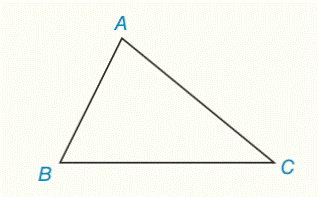Chapter 2.CR, Problem 41CR### Elementary Geometry for College St...

6th Edition
Daniel C. Alexander + 1 other
ISBN: 9781285195698

#### Solutions

Chapter
Section### Elementary Geometry for College St...

6th Edition
Daniel C. Alexander + 1 other
ISBN: 9781285195698
Textbook Problem
5 views

# Construct the line through C parallel to A B ¯ .To determine

To construct:

The line through C parallel to AB¯.

Explanation

Given:

A triangle ABC is given.

Figure (1)

Approach:

If two lines are cut by a transversal so that a pair of corresponding angles is congruent, then these lines are parallel.

Construction:

Step (1): Scribe an arbitrary arc on BC¯ from point B which intersect line BC¯ on point E and line AB¯ on point D.

Figure (2)

Step (2): Scribe an arc from point E which intersect AB¯ on point D

### Still sussing out bartleby?

Check out a sample textbook solution.

See a sample solution

#### The Solution to Your Study Problems

Bartleby provides explanations to thousands of textbook problems written by our experts, many with advanced degrees!

Get Started